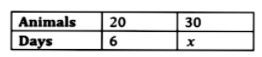Home/Class 8/Maths/

A farmer has enough food to feed $$20$$ animals in his cattle for $$6$$ days. How long would the food last if there were $$10$$ more animals in his cattle?Speed
00:00
02:09## QuestionMathsClass 8

A farmer has enough food to feed $$20$$ animals in his cattle for $$6$$ days. How long would the food last if there were $$10$$ more animals in his cattle?

$$4$$ days
4.64.6## Solution

Let the number of days be $$x$$.
Total number of animals $$= 20 + 10 = 30$$Here the number of animals and the number of days are in inverse proportion.
$$\implies \frac{20}{30}=\frac{x}{6}$$
$$\implies 30 \times x=20 \times 6$$
$$\implies x=\frac{20 \times 6}{30}$$
$$\implies x= 4$$
Hence the food will last for four days.